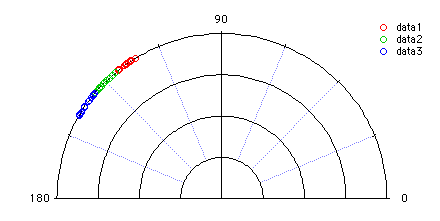In this example we have three groups of data contained in the numeric waves data1, data2 and data3. The values represent angles in radians:

 data1 data2 data3 2.173 2.34269 2.55387 2.15495 2.42743 2.52051 2.24984 2.35977 2.60009 2.16018 2.32347 2.60166 2.12517 2.34709 2.57084 2.17603 2.37079 2.47116 2.19694 2.27066 2.58406 2.17019 2.39049 2.4725 2.20484 2.36472 2.54683 2.24169 2.43949 2.46343 2.18406 2.26981 2.47491 2.24535 2.40376 2.53199 2.19074 2.40725 2.51363 2.18796 2.42927 2.54139 2.1496 2.29848 2.59919

The data are shown here in a polar plot:### Multi-sample testing for angular dispersion

The three input waves were created using uniformly distributed random numbers centered on 125, 135 and 145 degrees respectively. We therefore expect the angular distance test to indicate low dispersion. To run the test execute the command:
`  StatsAngularDistanceTest/T=1/Q/ANGM data1,data2,data3`

The results of the test are shown in the "Kruskal-Wallis Test" table:

 Num_Groups 3 Num_Valid_Data 45 Alpha 0.05 H 3.83614 Hc_Chi_square_approx 5.99146 P_Chi_square_approx 0.146891 Hc_Wallace_approx 6.04367 P_Wallace_approx 0.15194

The H statistic is clearly below both the Chi-square and Wallace approximate critical values so the hypothesis H0: no effective dispersion can't be rejected at the default 0.05 significance level. Note that in this case we have used the /ANGM flag to compute the relative dispersion of the data about each sample's mean instead of providing sample means using the /ANGW flag. Here the operation first computes the mean of each sample and then performs the Kruskal-Wallis test on the difference of each datum from its corresponding mean value.

### Two-sample testing for angular dispersion

Here we run a different test on the first two waves that we tested above. The operation computes the mean direction of the two samples and then performs the nonparametric Wilcoxon test on the differences between each datum and the mean direction. To run the test just execute the command:

`  StatsAngularDistanceTest/T=1/Q/ANGM data1,data2`

The results of the test are shown in the "Mann-Whitney Wilcoxon Test" table:

 m 15 n 15 TotalPoints 30 U_statistic 74 Up_statistic 151 P_Value 0.946777

As one would expect, we get a P-value which is much greater than alpha (0.05) so H0: no dispersion can't be rejected.

### Two-sample testing for angular dispersion with user-specified mean direction

In this example we test the same samples (which were generated as data centered at 125 and 135 degrees respectively) relative to mean direction of 135 degrees for both. To run the test execute the command:

`  StatsAngularDistanceTest/T=1/Q/ANG={2.35619,2.35619} data1,data2`

The results of the test are shown in the "Mann-Whitney Wilcoxon Test" table:

 m 15 n 15 totalPoints 30 U_statistic 225 Up_statistic 0 P_Value 6.38465e-09

Clearly, the P-value is less than alpha and so we reject H0.ForumSupportGallery